# Statistics Assignment 1

Very happy with the high mark I achieved for this first assignment. Though as usual, there's a decent about to be improving. Let's start by looking at some of the more major things my tutor pointed out.

# Variance

First thing is variance. How do you calculate it? Well it turns out there are a couple of ways. I just decided to use the most cumbersome way...

Calculating the mean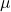(expectation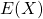) is easy. Multiply each number with its probability and sum them all: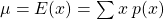The variance can then be calculated in one of two different ways: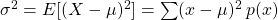or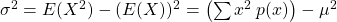When they're written out like this, it's fairly obvious to see which method is more like the method used to calculate the mean and as  such would be far less hassle. (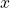is an integer and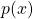andcan be reals/rationals).

# Multivariate Poisson Process

This was the question in which I lost the most marks:

Customers arrive at a shoe shop according to a Poisson process with a rate of 20 per hour.
15% of customers buy men's shoes.
Calculate the probability that exactly eight customers arrive in half an hour, exactly three of whom wish to purchase children's shoes.

This is such a typical mistake for me to make in statistics. I'm sure I've made this kind of mistake before...

What I ended up doing was working out the probability of the number of customers being 8 using the Poisson distribution's probability function. This was fine.

Then I used the same probability function to find the probability of 3 people wanting to buy children's shoes and multiplied them together. Wrong. At this point I needed to find the conditional probability that of the 8 customers, 3 bought children's shoes. Hence, here I shouldn't have used the Poisson distribution, I should've used the Binomial distribution instead. ie: from 8, choose 25%.

Reflecting back on the question, the correct answer seems slightly more obvious now. Especially given the "...exactly three of whom..." part of the question. I struggle to be mindful of stuff like this in the moment of answering a stats question. I suppose this part of the "translating English into maths" issue comes with more practise...

# Index Of Dispersion

Again, my issue here was to not observe subtleties in the question. Given information about the associated distributions, I was initially meant to calculate the mean and variance of the total number of books bought in 9 hours. I managed to get this first part right, but the second part of the question asked me to calculate the index of dispersion for "this process". It turns out that "this process" refers to the process in the main question generally and not the process of books being bought within 9 hours. In this instance, ignoring the total number of books bought in 9 hours (kind of) simplifies the answer too.

# Other Issues

In this first assignment, I lost a half a mark here and there for incorrect arithmetic. (GASP!). Upon completing my draft submission, instead of just reading through it, I should sit down and verify all my working. It will take more time, but if it scrapes 2 marks back, it could be worth it.

Other issue that occurred more than once was a lack of units when talking about rates of things happening. So there's a requirement to state "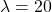per hour" instead of just "".